• python提取图片曲线坐标
2022-01-13 19:13:50

## python鼠标实时点击图片输出曲线上点的坐标

这里和大家分享一个简单的提取图片中曲线上点的坐标的小程序，程序依赖opencv2库。

import cv2

x_old = []
y_old = []
x_new = []
y_new = []
Ox,Oy = 0,0
e1 = 0 #x轴单位1对应的长度
e2 = 0 #y轴单位1对应的长度

def on_EVENT_LBUTTONDOWN(event, x, y, flags, param):
global Ox,Oy,e1,e2
if event == cv2.EVENT_LBUTTONDOWN:
if len(x_old) == 0:
Ox,Oy = x,y
x_old.append(x)
elif len(x_old) == 1:
e1 = x-Ox
x_old.append(x)
elif len(x_old) == 2:
e2 = y-Oy
x_old.append(x)
elif len(x_old) > 2:
x_ = (x-Ox)/e1
y_ = (y-Oy)/e2+9  #y轴上有个偏移
x_old.append(x)
y_old.append(y)
x_new.append(x_)
y_new.append(y_)
xy = "[%f,%f]" % (x_, y_)
cv2.circle(img, (x, y), 3, (255, 0, 0), thickness=-1)
cv2.putText(img, xy, (x, y), cv2.FONT_HERSHEY_PLAIN,
1.0, (107, 73, 251), thickness=1)	#如果不想在图片上显示坐标可以注释该行
cv2.imshow("image", img)
print("[{},{}], 原来坐标:[{},{}]".format(x_, y_, x_new[-1], y_new[-1]))	#终端中输出坐标

cv2.namedWindow("image")
cv2.setMouseCallback("image", on_EVENT_LBUTTONDOWN)
cv2.imshow("image", img)
cv2.waitKey(0)
cv2.destroyAllWindows()

with open('data.txt','w') as f:
for x,y in zip(x_new,y_new):
line = '{:<10f}\t{:<10f}\n'.format(x,y)
f.write(line)


运行该程序，然后：

1. 首先左键点击坐标系的坐标原点
2. 接着左键点击x坐标轴刻度1的点
3. 接着左键点击y坐标轴刻度1的点

然后就可以点击感兴趣的曲线上的点了，前面三次点击不会在图上标出。
效果如图所示：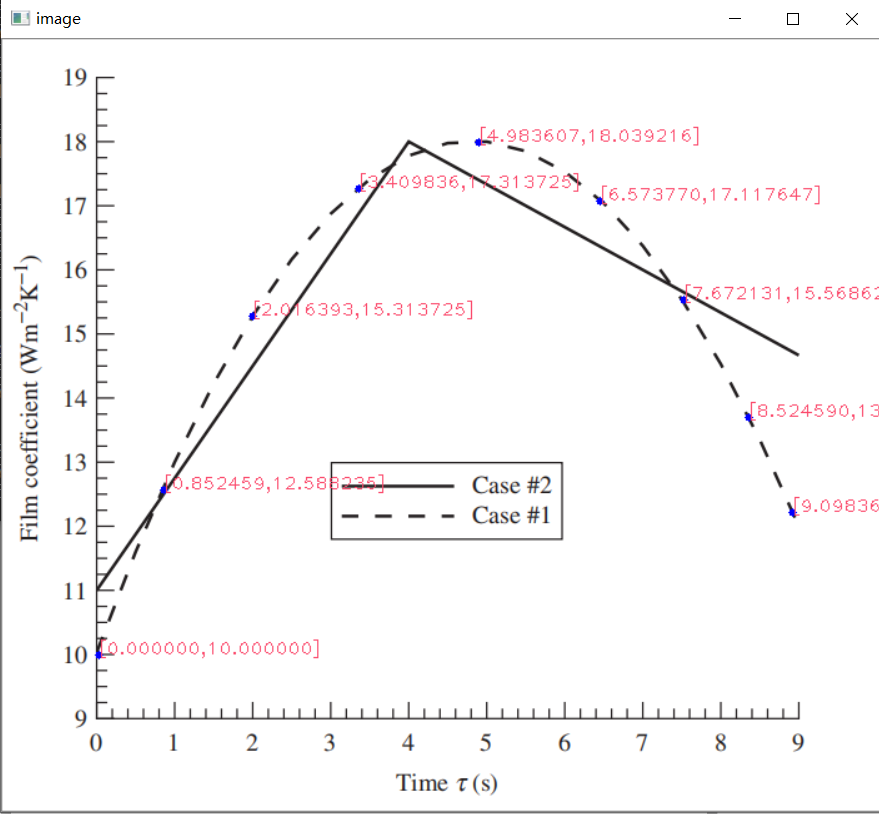同时会将数据写入data.txt中。

### Ref:opencv
更多相关内容
• 采用python，opencv开源库实现图片提取曲线数据 使用说明： openpic 可以选择采用自动采集测点数据或手动采集测点数据 操作过程：第一步裁剪,crop, 鼠标响应step_crop, 鼠标左键选裁剪的矩形区域，选中后键盘n表示...
• 此时，如果能从文中把这幅图截取下来，输入到一个函数中去，最后能返回从图片提取到的曲线坐标数据，岂不美哉。这便是本文的工作。1.思路详解与分析1.1准备待提取数据的曲线图片将待提取数据的曲线图片(如.jpg...

0.引言

在读文献的时，经常遇到这样的情况：文章里提出的方法好有趣啊，好想拿文中用的数据来试试看看能不能得到相近的结果，可是文中只有根据原始数据绘制的曲线图，没有数据。如下图所示。此时，如果能从文中把这幅图截取下来，输入到一个函数中去，最后能返回从图片中提取到的曲线的坐标数据，岂不美哉。

这便是本文的工作。

1.思路详解与分析

1.1准备待提取数据的曲线图片

1.2曲线图片预处理与数据转换

曲线图片预处理步骤的主要工作包含如下：

(1)图像二值化

将输入图像进行二值化处理，但分割得到的结果并不全为数据，其中可能还包括坐标轴等干扰点需要去除。

(2)获取从图片像素到曲线坐标的定标数据

首先，通过ginput()手动从图片中提取到两个像素点，这两个点分别为曲线坐标框的左上角和右下角。此时,便获得了曲线在图片上的像素范围

[x_index_min, x_index_max] & [y_index_min, y_index_max]

然后，手动输入实际曲线的数据坐标范围 [x_min, x_max] & [ymin, y_max]. 如下所示。此时，一方面得到了像素坐标，一方面得到了实际坐标。接下来便利用这对数据，将图片中全部的像素坐标转换到实际坐标。

最终，得到了由图片提取到的数据散点图，如下可以看到，此时得到的结果，虽然曲线与所需要的相近，但曲线外的部分，如坐标轴框、坐标轴刻度等也被转换成了数据，还需要进一步的处理。

1.3数据的进一步处理并得到最终曲线

这一步的主要工作是在数据中除去曲线之外的部分(包括坐标轴框、坐标轴刻度等)；以及解决一个x数据对应多个y数据的情况。

显然，坐标轴在整幅数据中，均处于边界位置，因此，很容易想到的一种方法是，设定阈值，将距离边界较近的数据直接删除。这里，设定了两个阈值，一个用来限定x方向上的数据，一个用来限定y方向上的数据。比如设定：rate_x=0.08; rate_y=0.05; 意思是阈值设定为曲线最前端8%和最后段8%的数据，曲线最顶端5%和最底端5%的数据。

进一步的，对于提取到的数据图，大多数情况一个x会对应若干y，因为数据是由图片转化而来，而图片的分辨率有限，一个实际数据点会用多个像素来表示。解决此问题的中心思想是将同一个x对应的若干个y取均值，但不能直接求均值，因为还有很多y是噪声(如坐标轴线、由图片噪声带入的干扰点等)需要先去除，在第一个问题中，通过限定y的范围，已经在一定程度上除去了部分干扰，在此基础上，我们求取一个x对应的这组y值的均值mean与标准差std，当某些y值位于[mean-std , mean+std]之外，则认为这些y值波动太大，将它们删去。

到这里，我们就将数据的处理部分基本完成了，我们将处理后的数据再次绘制成曲线，便可以得到如下对比处理之前的数据，由于限定了范围，因此曲线图片中带来的坐标框等内容转化而来的数据已经被删去。

将需要提取坐标的曲线图片，和我们提取并处理后的数据绘制的曲线，放在一起对比如下：可以看到，与原曲线图片相比，提取到的数据曲线相似度能达到较高要求。但进一步观察会发现，右图曲线较左图而言，高频分量有一定的减少(即右图曲线更平滑)，原因在于数据处理时，对同一个x对应的这组y值进行了均值处理，则在图像上近似反应为均值滤波，从而使得提取到的数据绘制成的曲线的高频分量被滤除。

最后，将提取到的最终数据，保存起来如下：1.4进一步的讨论——曲线拟合

通过对图片中曲线的数据提取，可以得到数值上的答案，这会带来进一步的思考，即能否得到这些数据的解析表达式。很容易想到，利用最小二乘法来拟合这些数据，这便涉及到了曲线的拟合。(插值与拟合可以这么理解：对于数据点集，若均落在曲线上，则该曲线为插值曲线，否则为拟合曲线)

对于一些简单的曲线图片(如下)，可以考虑用泰勒级数来近似，即多项式拟合。数据提取并拟合的结果展示如下同时还能得到拟合多项式的系数从而得到该曲线的多项式(这里采用四阶多项式)表达式为:理论上，泰勒级数可以分解任何函数，但实际上，多项式拟合的次数太高，会出现龙格库塔现象，即摆尾现象。因此，多项式拟合的阶数不易过高，一般低于5阶。对于本文最开始的那幅曲线而言，仅5阶的泰勒级数就显得力不从心了，因此，对于这种存在波动剧烈的函数，可以考虑用傅里叶级数进行拟合，或者如果能提供先验知识，可以直接用先验表达式进行拟合。

在MATLAB中，提供了cftool工具箱，其提供了拟合与插值的GUI，使用非常方便，直接在命令窗输入cftool即可调用，cftool界面如下所示，其具体使用方法不在此赘述。2.MATLAB程序

MATLAB源代码如下所示，和以往的风格一样，提供了详细的注释

% 提取图片中的曲线数据

clear,clc,close all

%% 图片与曲线间的定标

im=rgb2gray(im);%灰度变化

thresh = graythresh(im);%二值化阈值

im=im2bw(im,thresh);%二值化

set(0,'defaultfigurecolor','w')

imshow(im)%显示图片

[y,x]=find(im==0);%找出图形中的“黑点”的坐标。该坐标是一维数据。

y=max(y)-y;%将屏幕坐标转换为右手系笛卡尔坐标

y=fliplr(y);%fliplr()——左右翻转数组

plot(x,y,'r.','Markersize', 2);

disp('请在Figrure中先后点击实际坐标框的两个顶点(左上点和右下点)，即A、B两点. ');

[Xx,Yy]=ginput(2);%Xx,Yy——指实际坐标框的两个顶点

min_x=input('最小的x值');%输入x轴最小值

max_x=input('最大的x值');%输入x轴最大值

min_y=input('最小的y值');%输入y轴最小值

max_y=input('最大的y值');%输入y轴最大值

x=(x-Xx(1))*(max_x-min_x)/(Xx(2)-Xx(1))+min_x;

y=(y-Yy(1))*(min_y-max_y)/(Yy(2)-Yy(1))+max_y;

plot(x,y,'r.','Markersize', 2);

axis([min_x,max_x,min_y,max_y])%根据输入设置坐标范围

title('由原图片得到的未处理散点图')

%% 将散点转换为可用的曲线

%需处理的问题与解决思路

%(1)散点图中可能一个x对应好几个y 保留mean()-std()到mean()+std()之间的y值 并取平均处理

%(2)曲线的最前端和最后段干扰较大 去掉曲线整体的前(如5%)和后5%

%(3)曲线的最顶端和最底段干扰较大 去掉曲线整体的上10%和下10%

%参数预设

rate_x=0.08;%曲线的最前端和最后段删除比例

rate_y=0.05;%曲线的最顶端和最底段删除比例

[x_uni,index_x_uni]=unique(x);%找出有多少个不同的x坐标

x_uni(1:floor(length(x_uni)*rate_x))=[];%除去前rate_x(如5%)的x坐标

x_uni(floor(length(x_uni)*(1-rate_x)):end)=[];%除去后rate_x的x坐标

index_x_uni(1:floor(length(index_x_uni)*rate_x))=[];%除去前rate_x的x坐标

index_x_uni(floor(length(index_x_uni)*(1-rate_x)):end)=[];%除去后rate_x的x坐标

[mxu,~]=size(x_uni);

[mx,~]=size(x);

for ii=1:mxu

if ii==mxu

ytemp=y(index_x_uni(ii):mx);

else

ytemp=y(index_x_uni(ii):index_x_uni(ii+1));

end

%删除方差过大的异常点

threshold1=mean(ytemp)-std(ytemp);

threshold2=mean(ytemp)+std(ytemp);

ytemp(find(ytemp

ytemp(find(ytemp>threshold2))=[];

%删除距顶端和底端较近的点

thresholdy=(max_y-min_y)*rate_y;%y坐标向阈值

ytemp(find(ytemp>max_y-thresholdy))=[];%删除y轴向距离顶端与底端距离小于rate_y的坐标

ytemp(find(ytemp

%剩下的y求均值

y_uni(ii)=mean(ytemp);

end

%此时很多x_uni点处对应的y_uni为空,即NAN,要进一步删去这些空点

x_uni(find(isnan(y_uni)))=[];

y_uni(find(isnan(y_uni)))=[];

%画图

figure,plot(x_uni,y_uni),title('经处理后得到的扫描曲线')

axis([min_x,max_x,min_y,max_y])%根据输入设置坐标范围

% 将最终提取到的x与y数据保存

curve_val(1,:)=x_uni';

curve_val(2,:)=y_uni;

%% 对提取出的数据进行拟合(按实际情况进行修改)

[p,s]=polyfit(curve_val(1,:),curve_val(2,:),4);%多项式拟合(为避免龙格库塔,多项式拟合阶数不宜太高)

[y_fit,DELTA]=polyval(p,x_uni,s);%求拟合后多项式在x_uni对应的y_fit值

figure,plot(x_uni,y_fit),title('拟合后的曲线')

axis([min_x,max_x,min_y,max_y])%根据输入设置坐标范围

展开全文• 识别该图片中每隔0.01s的荷载数据，并导入到Excel中。 思路： 1. 预处理图片 2. 截取竖条 2.1 代码 import cv2 from PIL import Image import sys def cut_image(image): width, height = image.size print...

# 任务：

识别该图片中每隔0.01s的荷载数据，并导入到Excel中。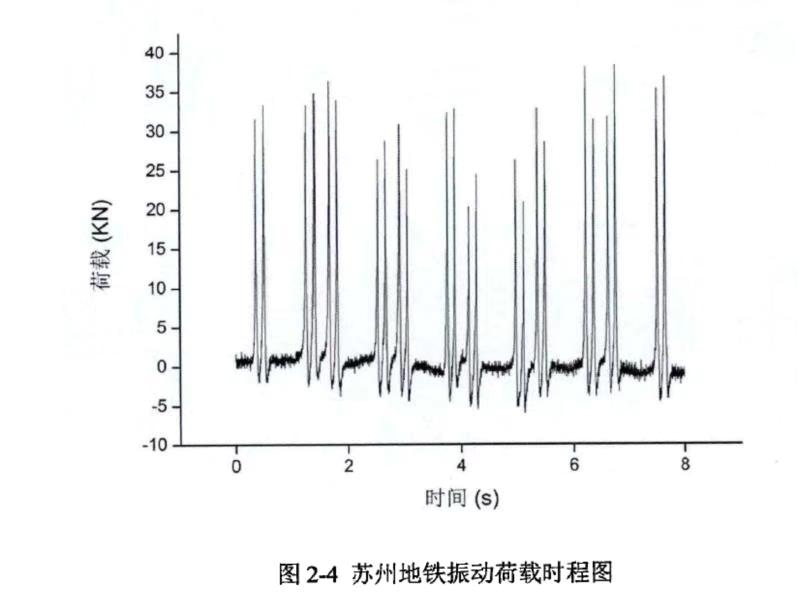# 思路：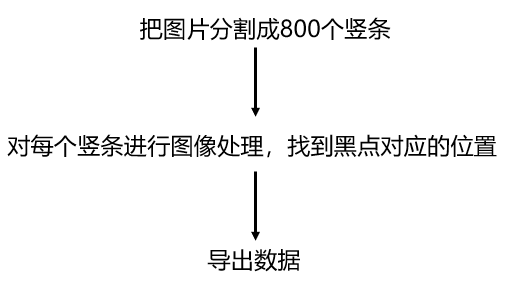## 1. 预处理图片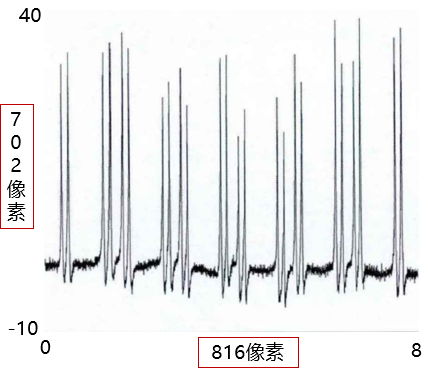## 2. 截取竖条

### 2.1 代码

import cv2
from PIL import Image
import sys

def cut_image(image):
width, height = image.size
print(width, height)
item_width = int(width / 800)
box_list = []
# (left, upper, right, lower)
for i in range(800):#0.01s为一段，8s=800个0.01s
box = (i*item_width,0,(i+1)*item_width,height)
box_list.append(box)

image_list = [image.crop(box) for box in box_list] #crop用于切割图片
return image_list

def save_images(image_list):
index = 1
for image in image_list:
image.save('D:/python_opencv/img/'+str(index) + '.png', 'PNG')
index += 1

if __name__ == '__main__':
file_path = "D:/002.png"
image = Image.open(file_path)
#image.show()
image_list = cut_image(image)
save_images(image_list)



### 2.2 效果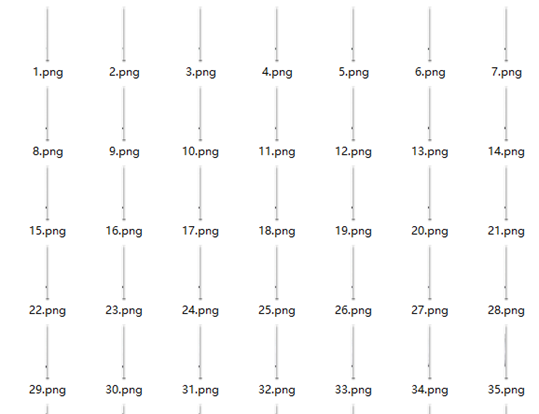## 3. 对每个竖条图片处理

### 3.1 代码

import cv2
import numpy as np
import shutil
import os
import math
import matplotlib.pyplot as plt

Img_FOLDER = "D:/python_opencv/img"

list_y = []

for i in range(800):
hsv = cv2.cvtColor(img, cv2.COLOR_BGR2HSV)
low_hsv = np.array([0,0,46])
high_hsv = np.array([180,255,220])
yx = 702-yx
y = max(yx[:,0])
y_new = y*50/702 - 10  #根据像素与所需尺度的关系确定
list_y.append(y_new)

list_x = np.arange(0,8,0.01)

plt.plot(list_x,list_y,label='Frist line',linewidth=3,color='r')
plt.show()

cv2.waitKey(0)
cv2.destroyAllWindows()



### 3.2 效果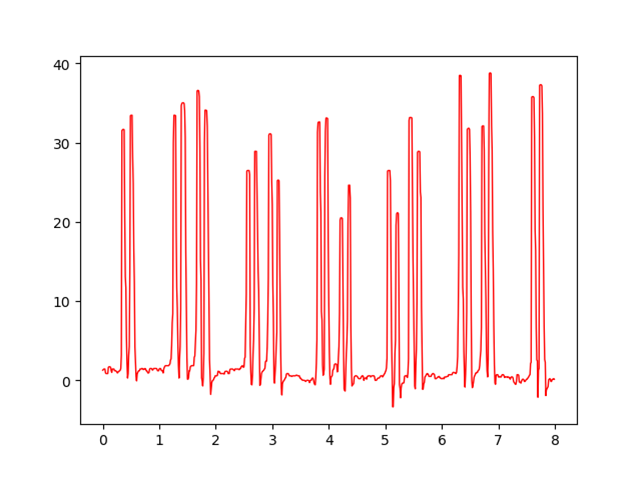## 4. 导出

### 4.1 代码

import xlwt
wb = xlwt.Workbook()
# 添加一个表

# 3个参数分别为行号，列号，和内容
# 需要注意的是行号和列号都是从0开始的

ws.write(0, 0, "x")
ws.write(0, 1, "y")
i = 1
for x in list_x:
ws.write(i, 0, x)
i += 1

j = 1
for y in list_y:
ws.write(j, 1, y)
j += 1

# 保存excel文件
wb.save('D:/python_opencv/result.xls')


### 4.2 效果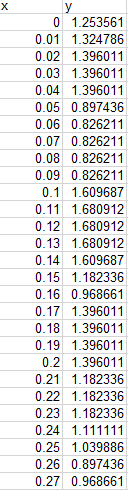展开全文• 设定灰度值阈值，输出较大的像素灰度值坐标——像素坐标系，转存入TXT文件中
• 本文主要讲述利用python接口的opencv来完成图像识别和信息提取并重新绘制、保存为excel数据的详细过程与思路，适合opencv方面的小白观看

# 编程实战（4）——python识别图像中的坐标点并保存坐标数据

### 综述

最近因为美赛的需求，需要在提取一些赛道的路线图和地形图中的准确数据，因此对这方面做了一些了解。在研究的过程中，我发现网上的很多相关的帖子并不是很靠谱，不是报错就是没有说清楚一些函数的功能，所以我打算写一篇比较详细的文章。

本文主要讲述利用python接口的opencv来完成图像识别和信息提取并重新绘制、保存为excel数据的详细过程与思路，适合opencv方面的小白观看（需要一定的numpy和matplotlib基础）。如有一些疏漏，请大佬们指出~

### 代码思路

我会跟着我代码的思路逐一讲解每一步的思路和函数的一些解释；

总体思路如下：

• 第一：图片预处理，让图像二极化；
• 第二：提取图片数据
• 第三步：数据整理与保存

#### 库的安装

这里一共用了三个库

import cv2
import numpy as np
import matplotlib.pyplot as plt


numpy和matplotlib的安装都比较常规，但是cv2的安装不是常规的pip install cv2，是opencv-python，国内镜像下载地址：

pip3 install -i https://mirrors.aliyun.com/pypi/simple/ opencv-python


#### 图片预处理

img = cv2.imread('你的图片路径')
hsv = cv2.cvtColor(img, cv2.COLOR_BGR2HSV)


首先打开图片，然后对图片做一个hsv灰度图的处理，因为我们常规的图片像素点都是rbg空间模式的，我们需要先转化为hsv颜色空间模式（没见过这个图像格式的可以百度一下），以便后面的图像二值化处理；上面是我用到的图像，这是东京奥运会公路自行车比赛的路线图，我们要做的就是提取路线像素并坐标化；

##### 图像细化

如果说要处理的图像的线条很粗，那就会影响后面的识别过程，需要先进行图像细化；如果原本图的线条就很细（比如我的），那就可以跳过这一步。

图像细化我是直接参考一个博客的代码的，这里做一个引用：图像细化，骨架提取

写的相当棒，大家可以参考一下。

##### 图像二极化

这里我用到了一个cv库中的inRange函数，这个函数的功能是对读入的图像文件（即函数第一个参数）做一个二值化处理。

总的来说就是我们需要规定两个阈值lowerb和upperb，大于upperb和小于lowerb的图像像素点均会被转化为0（即黑色），在这个范围内的点被转化为255（白色）；

low_hsv = np.array([0, 0, 221])
high_hsv = np.array([180, 30, 255])


我们再来详细的讲一下这两个阈值，如果了解hsv颜色空间的话，很容易可以发现代码的前两行就是hsv颜色空间的两个值，numpy.array函数里面的参数刚好就是色调、饱和度和明度值，因为inRange函数的需要，我们要转化成numpy格式的数组。我这里需要提取所有黑色的坐标，所以设的是黑色与白色的阈值，大家可以根据自己的需求调整颜色阈值。

阈值参考：HSV基本颜色分量范围

#### 提取数据

print(len(mask))


输出：

654
1024
[255 255 255 ... 255 255 255]
[255 255 255 ... 255 255 255]
...
[255 255 255 ... 255 255 255]
[255 255 255 ... 255 255 255]
[255 255 255 ... 255 255 255]


可以看到图像是1024*654的，还有每一行的像素点都是一个numpy数组（numpy数组打印出来元素之间是没有用逗号间隔的），之前24位的hsv图，变成了8位的灰度图（每一项只有一个数据，而不是三个数组成的array）；

方法也很简单，直接遍历每一个像素，找到值为0的像素点，存取列号和行号即可；

list_y = []
list_x = []

xmax = []
list_x.append(j)


#### 结果展示和保存

##### matplotlib重绘

检验一下我们获取的图像数据，注意这里需要用散点图模式绘图，不然会有不太好的后果。。。

plt.plot(list_x, list_y, 'o', color='r')
plt.show()


结果如下：可以发现提取效果还是不错的~~

##### 写入excel

这一部分的说明直接放到注释里面了

import xlwt

wb = xlwt.Workbook()

ws.write(0, 0, "x") # 写入数据，3个参数分别为行号，列号，和内容
ws.write(0, 1, "y")

i = 1 #指针，每写一个数据，向下移动写指针一行
for x in list_x:
ws.write(i, 0, x)
i += 1

j = 1
for y in list_y:
ws.write(j, 1, y)
j += 1

wb.save('1111.xls')


可以看到数据已经保存进excel里面了这一次提取其实还有一个小问题，就是相同的横坐标下还是有多个对应该横坐标的点，如果需要做函数分析之类的操作，我们可以直接用excel作按值分组然后去每组的特定值即可，最大值，平均值均可，看个人需求。

### 全部源码

import cv2
import numpy as np
import matplotlib.pyplot as plt
import xlwt

hsv = cv2.cvtColor(img, cv2.COLOR_BGR2HSV)
low_hsv = np.array([0, 0, 221])
high_hsv = np.array([180, 30, 255])

list_y = []
list_x = []

xmax = []
list_x.append(j)

plt.plot(list_x, list_y, 'o', color='r')
plt.show()

wb = xlwt.Workbook()

ws.write(0, 0, "x")
ws.write(0, 1, "y")
i = 1
for x in list_x:
ws.write(i, 0, x)
i += 1

j = 1
for y in list_y:
ws.write(j, 1, y)
j += 1

wb.save('1111.xls')

展开全文计算机视觉 opencv
• #绘制某省新冠疫情曲线Python源码 1. 使用pandas库读取本地.csv文件； 2. 从读取的数据中提取某省份数据； 3. 使用matplotlib库绘制曲线图； 4. 将绘制完成的图片显示并保存。
• Python+OpenCV图像处理之直线检测_天为我蓝的博客-CSDN博客霍夫变换是图像处理中从图像中识别几何形状的基本方法之一，应用很广泛，也有很多改进算法。主要用来从图像中分离出具有某种相同特征的几何形状（如，直线...opencv
• import numpy as np import matplotlib.pyplot as plt import cv2 import pylab as mpl # import matplotlib as mpl from scipy....y # plt.plot(sav_y) # plt.show() 原图片长这样，看看有没有搞得更好 我处理出来的计算机视觉
• 目录1. 前言2. 数据提取2.1 图像预处理2.2 提取...利用画图对原始图像进行简单处理，去除外围的边框线和内部的网格线，保留曲线部分以及坐标轴的边界线，方便后续数据提取： 2.2 提取数据 导入要用的模块和包 import
• returnPoints:设置为False会返回与凸包上对应的轮廓的点索引值，设置为True，则会返回凸包上的点坐标集，默认为True 对于opencv-python提取图像轮廓部分有问题欢迎留言， Have Fun With OpenCV-Python, 下期见。
• 提取条件： （1）刻度线方向朝内，长度为6，宽度为2，标签大小为12 （2）x轴刻度标签为固定格式的时间 下面是完整代码以及注释： dates = ['201910240', '2019102402', '2019102404', '201910240.matplotlib
• import easyocr #识别图片上的数字/数值/文字方法 from PIL import Image #打开图片，传输图片信息 import re #正则表达式 # import pytesseract #识别图片上的数字/数值方法 应用场景：单独识别图片中某个区域的...开发语言
• 目录Python数据处理从零开始----第四章(可视化)⑨线性相关曲线==============================================================================================通过python我们可以绘制两个变量的相关图，我所使用...
• python绘制训练结果曲线图和散点图、解决坐标刻度标签重复问题 、利用训练标准输出流绘制python绘制训练结果图训练结果标准输出流删除标准输出流中多余内容正则表达式提取数据绘制曲线图和散点图戏剧性bug，y轴标签...excel
• 前言：在前一篇关于PyCharm中遇到exit code -1073740791 (0xC0000409)的问题及其解决方法中有提到将图片中的曲线提取出来，并使用神经网络进行曲线拟合，第一步实现了，但神经网络拟合效果始终不理想（可能是选择的...神经网络 图像识别
• 现在，人们已经养成了通过网络进行数据文件通信的习惯，方便的同时，数据和图片也会受到黑客之类的恶意攻击，造成数据错误、误传、篡改。随着网络密码技术的出现，网络的安全得到了很大的保护，以 DES为基础的对称...算法
• 利用Python绘制科研论文折线图，通过读取exal大量数据，进行折线图绘制。机器学习
• 特征提取的结果是把图像上的点分为不同的子集，这些子集往往属于孤立的点、连续的曲线或者连续的区域。 那么，什么样的点才能称之为特征呢？ 至今为止特征并没有绝对精确的定义。特征的精确定义往往由问题或者应用...计算机视觉
• 使用Python抽取PDF文件内容，包括文本、图像、线条等对象摘要：这篇文章主要介绍如何使用Python【3.6版本】中的PDFminer3k 模块来抽取PDF内容，包括文本、图像、曲线等。1.安装PDFminer3k使用pip 命令安装pip ...
• 计算图像中最长与最短曲线，并获取其重心坐标。 代码： import cv2 import numpy as np def calc_coordinate_area(contour): M = cv2.moments(contour) # 计算轮廓的各阶矩,字典 # print(M) # 计算重心 cx = ...opencv
• (图形来源：Microsoft Office专业增强版2019图图库)细心的伙伴不难发现上图的每个图形外面都画了一圈黑色虚线，不错，这些虚线就是根据图形轮廓提取的图像坐标绘制的。之前有伙伴...opencv 计算机视觉 matlab 人工智能opencv
• matlab处理图像数据，获取曲线对应的xy坐标
• 后面需要将相应算法翻译到C++环境中，而Skimage没有对应的C++版本，为了确保python算法和C++算法结果的一致性，需要进行迁移，因而打算使用OpenCV来重写去除孤立小区域的代码。代码如下： _,binary = cv2....
• 这篇博客将介绍如何使用Python，OpenCV进行基本的图像处理——提取红色圆圈轮廓并绘制。opencv 深度学习 图像处理 轮廓检测
• 特征提取Python中，一个单声道10kHz的波形文件表示为一个范围为-254到255的整数列表，每秒声音包含10000个整数。每个整数代表歌曲在对应时间点上的相对幅度。我们将分别从两首歌曲中分别提取一段时长60秒的片段......